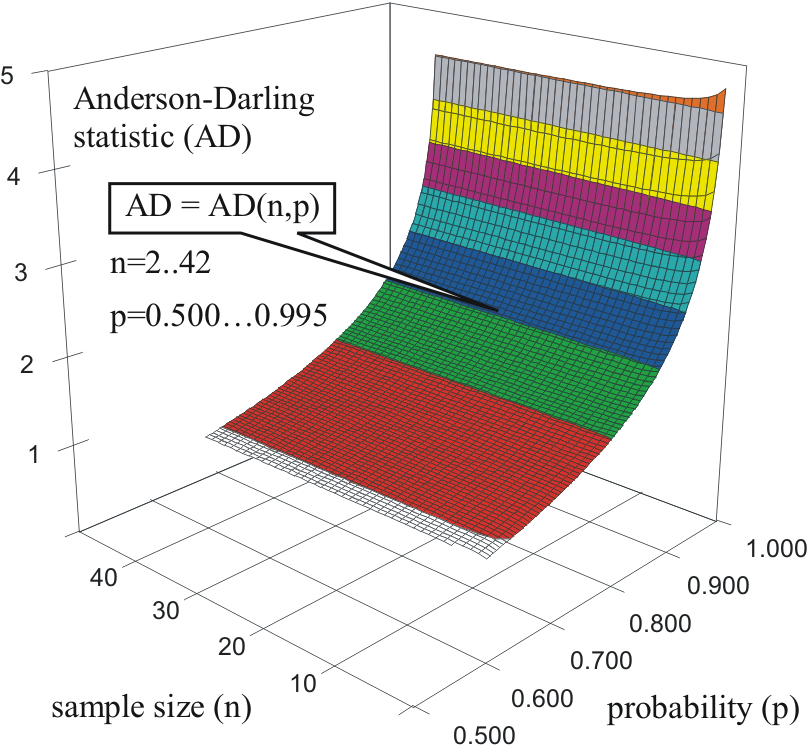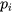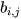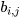Others

# Probability Associated with Anderson-Darling Statistic

Subjects: Probability and Statistics View times: 552

The subject of this entry is on the regard of one of the order statistics: the Anderson-Darling statistic.

We provided a method of calculation for the probability associated with the Cumulative Density Function (CDF) of the Anderson-Darling statistic.

Our study shown that the value of the probability is affected by the sample size. As consequence, we constructed a function to provide an estimate for the associated probability depending on both the value of the statistic and the sample size.A commonly known fact among all order statistics is that is very difficult to extract the probability associated with the statistic for a simple fact: their CDF (cumulative density function) - depicted in the figure for a range of probabilities from 0.500 to 0.995 (with a step of 0.5%) and for a range of sample sizes from n = 2 to n = 42 - have no analytical expression (e.g. we do not possess a mathematical function which to express it analytically). Unfortunately, the same case applies for their PDF (probability density function) making unavailable also the numeric integration methods. The only way of associating the probability (e.g., α = 1 - p) with the statistic is from Monte-Carlo experiments, and this is the way in which all were reported (see ,  and  for instance) and are on the use today.

Unfortunately, it is an inconvenience to use the raw data from Monte-Carlo simulations, namely we may have access to certain thresholds (for instance the value of the statistic corresponding to α = 5%), but it is not possible to extract the probability associated with a particular value of the statistic (for a simple reason: it is not tabulated). For some instances, when the statistic it is in range of tabulated data, the interpolation may provide satisfactory results - but also here precautions should be taken because the probability does not vary linearly with the statistic.

We run a computationally Monte-Carlo simulation with the Anderson-Darling statistic (named AD in the next) big and precise enough to obtain a good estimate of the probability associated with the statistic. The simulation strategy, the estimation strategy and the obtained function expressing the probability associated with the AD statistic are given in .

$\hat{p} = \Bigg( \sum_{i=0}^{4}{\sum_{j=0}^{4}{b_{i,j}*x^{i/4}}*n^{-j}} \Bigg)^{-1}, x = e^{AD}$

where AD is the calculated Anderson-Darling statistic on the sample of size n:

$AD = - n - \sum_{i=1}^{n}{ \frac{(2 \cdot i-1)\cdot ln(p_{i} \cdot (1-p_{n+1-i}))}{n} }$

andis the ordered (ascending) probability measuring that i-th (ordered) observation to belong to a certain distribution.

The values for thecoefficients are given in the following table.j = 0 j = 1 j = 2 j =3 j = 4 i = 0 5.6737 -38.9087 88.7461 -179.5470 199.3247 i = 1 -13.5729 83.6500 -181.6768 347.6606 -367.4883 i = 2 12.0750 -70.3770 139.8035 -245.6051 243.5784 i = 3 -7.3190 30.4792 -49.9105 76.7476 -70.1764 i = 4 3.7309 -6.1885 7.3420 -9.3021 7.7018

The proposed model is intended to be used (the applicability domain is) when p > 0.5 (e.g., α < 0.5).

The AD statistic (as any other order statistic) can be used to test (to measure the agreement) any distribution, not only the well-known normal (Gauss) one.

The use of AD statistic requires an estimate of the parameters of the distribution being tested. Certain precautions should be made, namely if the estimation of (some of) the parameters are not made with Maximum Likelihood Estimation method (see ; the usually alternative being the Central Moments method, see ), then a corresponding number must be subtracted from sample size value before to extract the probability associated with the statistic.

## References

1. Kolmogorov, A Sulla determinazione empirica di una legge di distribuzione. Giornale dell'Istituto Italiano degli Attuari 1933, 4, 83-91, N.A..
2. Smirnov, N. Table for estimating the goodness of fit of empirical distributions. Annals of Mathematical Statistics 1948, 19, 279-281, 10.1214/aoms/1177730256.
3. Anderson, T.W.; Darling, D.A. Asymptotic theory of certain "goodness-of-fit" criteria based on stochastic processes. Annals of Mathematical Statistics 1952, 23, 193-212, 10.1214/aoms/1177729437.
4. Anderson, T.W.; Darling, D.A. A Test of Goodness-of-Fit. Journal of the American Statistical Association 1954, 49, 765-769, 10.2307/2281537.
5. Jäntschi, L.; Bolboacă S.D. Computation of probability associated with Anderson-Darling statistic. Mathematics 2018, 6(6), 88, 10.3390/math6060088.
6. Fisher, R.A. On an Absolute Criterion for Fitting Frequency Curves. Messenger of Mathematics 1912, 41, 155-160, N.A..
7. Fisher, R.A. On the Mathematical Foundations of Theoretical Statistics. Philosophical Transactions of the Royal Society A 1922, 222, 309-368, 10.1098/rsta.1922.0009.